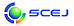## \$B9V1i%W%m%0%i%`!J%;%C%7%g%sJL!K(B

English page

### \$BD6NW3&N.BN(B

#### N113-N116, N213-N225

\$B:G=*99?7F|;~!'(B2016-02-26 18:17:04
\$BMW;]\$r8x3+\$7\$^\$7\$?!#9V1iHV9f\$r%/%j%C%/\$7\$F\$/\$@\$5\$\$!#(B
\$B9V1i(B
\$B;~9o(B
\$B9V1i(B
\$BHV9f(B
\$B9V1iBjL\!?H/I=\$B%-!<%o!<%I(B\$BJ,N`(B
\$BHV9f(B
\$B\$BHV9f(B
N \$B2q>l!&Bh(B 1 \$BF|(B
(13:00\$B!A(B14:20) (\$B:BD9(B \$B2<;3(B \$BM52p!&FbED(B \$BGn5W(B)
13:00\$B!A(B 13:20N113\$B9b299b05%"%k%3!<%k?eMO1U\$NFCD'E*\$JL)EY!&G4EY5sF0\$N2r@O\$*\$h\$SAj4X
(\$BElKLBg1!9)(B) (\$B@5(B)\$B!{>.Ln(B \$B9*(B\$B!&(B (\$B3X(B·\$B5;4p(B)\$B1+_7(B \$BN<2p(B\$B!&(B (\$BElKLBg9)(B) (\$B3X(B·\$B5;4p(B)\$B7PED(B \$BK((B\$B!&(B (\$BElKLBg1!9)(B) (\$B@5(B)\$BBgED(B \$B>;\$B!&(B (\$B@5(B)\$B:4F#(B \$BA1G7(B\$B!&(B (\$B@5(B)\$BCv8T(B \$B9((B
alcohol-water mixture
density
viscosity
8-b771
13:20\$B!A(B 13:40N114Extraction of Phenolic Compounds from Garlic Husk Using Carbon Dioxide Expanded Ethanol
(\$BL>Bg1!9)(B) (\$B3X(B)\$B!{%A%g!<%/(B \$B%-%`%F%C%H(B\$B!&(B (\$B3X(B)\$B>e?9(B \$B@iJf(B\$B!&(B (\$B@5(B)Wahyudiono\$B!&(B (\$B@5(B)\$B?@ED(B \$B1Q51(B\$B!&(B (\$B@5(B)\$B8eF#(B \$B85?.(B
phenolic compounds
carbon dioxide expanded ethanol
extraction
8-c254
13:40\$B!A(B 14:00N115\$B<>=a>uBV\$N7>At\$H1_@PAt\$+\$i\$N1U2=%8%a%A%k%(!<%F%k\$K\$h\$kL};i\$ND>@\Cj=P(B
(\$BL>Bg1!9)(B/JST\$B\$5\$-\$,\$1(B/JST-JICA SATREPS) (\$B@5(B)\$B!{?@ED(B \$B1Q51!&(B (\$BL>Bg1!9)(B) (\$B3X(B)\$B@1Ln(B \$BNQB@O/(B\$B!&(B (\$B3X(B)\$BB<>e(B \$BOBLo(B\$B!&(B (\$B@5(B)Wahyudiono\$B!&(B (\$B@5(B)\$B8eF#(B \$B85?.(B
Extraction
Dimethyl ether
Microalgae
8-c471
14:00\$B!A(B 14:20N116Extraction and micronization of beta-glucan from barley by mixture of subcritical water and supercritical carbon dioxide
(\$BL>Bg1!9)(B) (\$B@5(B)\$B!{(BWahyudiono\$B!&(B (\$B3X(B)\$B;y6L(B \$B>M;R(B\$B!&(B (\$B@5(B)\$B?@ED(B \$B1Q51(B\$B!&(B (\$B@5(B)\$B8eF#(B \$B85?.(B
beta-glucan
extraction
micronization
8-c637
N \$B2q>l!&Bh(B 2 \$BF|(B
(13:00\$B!A(B14:40) (\$B:BD9(B \$B>.Ln(B \$B9*(B)
13:00\$B!A(B 13:20N213\$BD6NW3&N.BN\$rMQ\$\$\$??7\$7\$\$B?9&
(\$BBg:e%,%9%1%_%+%k(B) (\$B@5(B)\$B8b(B \$BFo(B\$B!&(B (\$B@5(B)\$BBgKL(B \$B@5?.(B\$B!&(B (\$B%@%\$%@%s(B) (\$B@5(B)\$BCfLn(B \$B0l\$B!&(B (\$B1'ET5\Bg9)(B) (\$B@5(B)\$B!{hSED(B \$B??><(B
Supercritical CO2
Active carbon
Pore size control
8-e275
13:20\$B!A(B 13:40N214\$BD6NW3&Fs;@2=C:AG7O\$NAj5sF0\$rMxMQ\$7\$?B?9&
(\$BEl9)Bg1!M}9)(B) (\$B@5(B)\$B!{2<;3(B \$BM52p(B\$B!&(B (\$B3X(B)Kunanusont Nattanai\$B!&(B (\$B3X(B)\$BLZ2<(B \$B85Bg(B
supercritical carbon dioxide
porous material
phase behavior
8-e863
13:40\$B!A(B 14:00N215\$BD6NW3&N.BN\$K\$h\$j7A@.\$7\$?(BRuO2\$BGvKl\$N7k>=2=\$K8~\$1\$?%"%K!<%k=hM}(B
(\$BElBg1!9)(B) (\$B3X(B)\$B!{%A%g%&(B \$B%f%&(B\$B!&(B (\$B@5(B)\$B2<;3(B \$BM52p(B\$B!&(B (\$B@5(B)\$BI4@%(B \$B7r(B\$B!&(B (\$B@5(B)\$BAz3@(B \$B9,9@(B
supercritical fluid
anneal
RuO2
8-e507
14:00\$B!A(B 14:20N216\$BD6NW3&Fs;@2=C:AG\$rMxMQ\$7\$?%U%CAG7O%]%j%\$%_%I\$N>xCe=E9g(B
(\$B9-Bg1!9)(B) (\$B@5(B)\$B!{=ULZ(B \$B>-;J(B\$B!&(B (\$B3X(B·\$B5;4p(B)\$B>.ED(B \$BL@F|9a(B\$B!&(B (\$B3X(B·\$B5;4p(B)\$BOB:4ED(B \$B29I'(B\$B!&(B (\$B@5(B)\$BLZ86(B \$B?-0l(B\$B!&(B (\$B@5(B)\$BBlV:(B \$BHK
Polyimide
Deposition polymerization
Supercritical carbon dioxide
8-e543
14:20\$B!A(B 14:40N217\$B@{2sN.7?%^%\$%/%m%_%-%5!<\$rMQ\$\$\$?D6NW3&IOMOG^2=K!\$K\$h\$k%"%;%H%"%_%N%U%'%s\$NHyN3;R2=(B
(\$BL>Bg1!9)(B) (\$B3X(B)\$B!{>e?9(B \$B@iJf(B\$B!&(B (\$B@5(B)Wahyudiono\$B!&(B (\$B%9%i%P%d9)Bg(B) (\$B3\$(B)Machmudah Siti\$B!&(B (\$BL>Bg1!9)(B) (\$B@5(B)\$B?@ED(B \$B1Q51(B\$B!&(B (\$B@5(B)\$B8eF#(B \$B85?.(B
anti-solvent
supercritical fluid
swallow micro-mixer
8-e815

(15:00\$B!A(B16:20) (\$B:BD9(B \$BLZ86(B \$B?-0l(B)
15:00\$B!A(B 15:20N219\$BD6NW3&?eG.K!\$K\$h\$j9g@.\$7\$?J#9g;@2=J*%J%NN3;R\$N7g4Y9=B\$(B
(\$BElBg1!?7NN0h(B) (\$B3X(B)\$B!{2#(B \$BE/(B\$B!&(B (NIMS) \$BG__7(B \$BD>?M(B\$B!&(B \$BBgLn(B \$BN41{(B\$B!&(B (\$BElBg1!?7NN0h(B) (\$B@5(B)\$BBgEg(B \$B5A?M(B
Defect
XAFS
First-principles calculation
8-e374
15:20\$B!A(B 15:40N220\$BD6NW3&Fs;@2=C:AGCf\$G\$N7V8w@-(BZnO\$BNL;R%I%C%H%J%NN3;R\$NJ#9g2=(B
(\$B5WN1JF9b@l(B) (\$B@5(B)\$B!{>>;3(B \$B@6(B\$B!&(B \$BA0ED(B \$BM&5.(B\$B!&(B \$B1|;3(B \$BE/Li(B\$B!&(B (\$BK-665;Bg(B) \$BIpF#(B \$B9@9T(B
Supercritical CO2
ZnO quantum dot
Poly(methyl methacrylate)
8-e625
15:40\$B!A(B 16:00N221\$B%l!<%6!<%"%V%l!<%7%g%sK!\$K\$h\$j@8@.\$9\$kC:AG%J%NN3;R\$N7ABV\$K5Z\$\\$9J70O5\$N.BN\$N1F6A(B
(\$B6bBtBgM}9)(B) (\$B@5(B)\$B!{El(B \$B=(7{(B\$B!&(B (\$B6bBtBg<+A38&(B) \$B5H@n(B \$BE0(B\$B!&(B (\$B6bBtBgM}9)(B) (\$B@5(B)\$B5bED(B \$B44IW(B\$B!&(B (\$B@5(B)\$B@%8M(B \$B>OJ8(B\$B!&(B (\$B@5(B)\$BBgC+(B \$B5H@8(B
laser ablation
carbon
nanoparticle
8-e654
16:00\$B!A(B 16:20N222Functionalization and Characterization of Graphene Oxide in Supercritical Aqueous Ammonia
(De La Salle U.) (\$B3\$(B)\$B!{(BBaldovino Fritzie Hannah\$B!&(B (Kumamoto U.) (\$B@5(B)Quitain Armando T.\$B!&(B (De La Salle U.) Dugos Nathaniel\$B!&(B Roces Susan\$B!&(B (Kumamoto U.) (\$B@5(B)Kida Tetsuya
Graphene Oxide
Supercritical Ammonia
Functionalization
8-e828
(16:20\$B!A(B17:20) (\$B:BD9(B \$B?@ED(B \$B1Q51!&2,Eg(B \$B\$\$\$E\$_(B)
16:20\$B!A(B 16:40N223Catalytic Supercritical Hydrothermal Liquefaction of Malaysian Oil Palm Biomass to Bio-Oil
(Kumamoto U.) (\$B@5(B)\$B!{(BQuitain Armando T.\$B!&(B (Universiti Teknologi PETRONAS) Yim See Cheng\$B!&(B Yusup Suzana\$B!&(B (Kumamoto U.) (\$B@5(B)Sasaki Mitsuru\$B!&(B (Universiti Teknologi PETRONAS) (\$B@5(B)Uemura Yoshimitsu\$B!&(B (Kumamoto U.) (\$B@5(B)Kida Tetsuya
Supercritical Water
Catalysts
Bio-oil
8-f838
16:40\$B!A(B 17:00N224\$BD6NW3&?eCf\$N8GBN;@?(G^\$N0BDj@-\$KBP\$9\$k?e\$N8z2L(B
(\$BElBg1!?7NN0h(B) (\$B@5(B)\$B!{=)7n(B \$B?.(B\$B!&(B (\$B@5(B)\$BBgEg(B \$B5A?M(B
Supercritical water
Solid acid catalysts
Catalyst stability
8-d436
17:00\$B!A(B 17:20N225\$B9b299b05?e%^%\$%/%m2=3X%W%m%;%9\$K\$h\$k%X%F%m85AG4^M-K'9aB2J,;R\$N?WB.9g@.(B
(\$B;:Am8&(B) (\$B@5(B)\$B!{D9Hx(B \$B0i90(B\$B!&(B (\$B@5(B)\$B@P:d(B \$B9'G7(B\$B!&(B (\$B@5(B)\$B@nGH(B \$BH%(B
High Pressure High Temperature Water
Micro Flow Process
Organic Synthesis
8-d395

\$B9V1iH/I=%W%m%0%i%`(B
\$B2=3X9)3X2q(B \$BBh(B81\$BG/2q(B (2016)(C) 2016 \$B8x1W
Most recent update: 2016-02-26 18:17:04
E-mail: inquiry-81awww3.scej.org
This page was generated byeasp 2.39; proghtml 2.37 (C)1999-2015 kawase